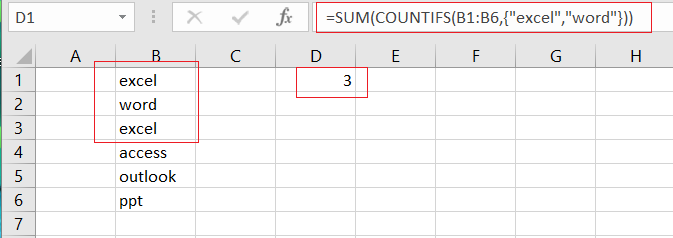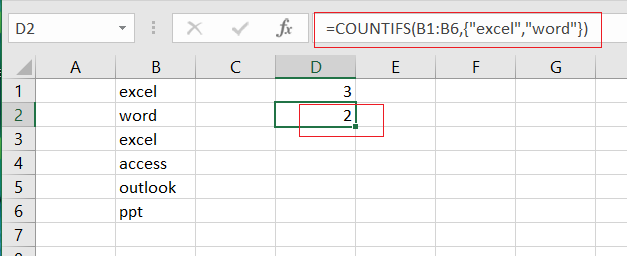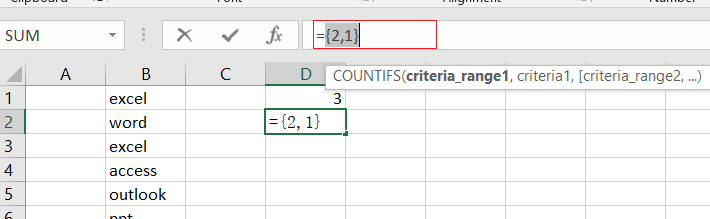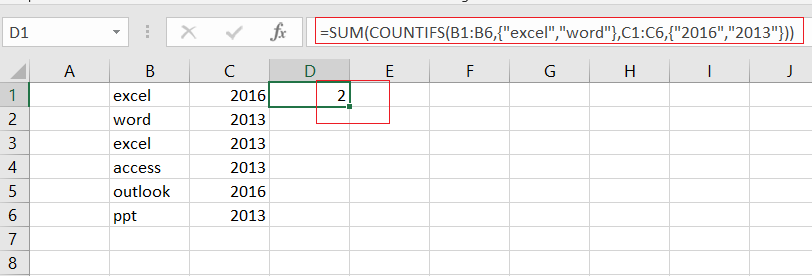# How to Use COUNTIFS function with Multiple Criteria and OR Logic in Excel

This post will guide you how to Use COUNTIFS function with multiple criteria and OR logic in Excel 2013/2016 or Excel office 365. How to count with multiple criteria and OR logic in Excel.

## COUNTIFS with Multiple Criteria and OR Logic

Normally, when you provide multiple conditions to a COUNTIFS function, and it will check all the conditions and returns rows which matches all the conditions, it means that it runs on AND logic.

If you want to count with multiple conditions and OR logic in your formula, and you can also use the COUNTIFS function to achieve OR logic. Then you still need to use the SUM function and array with multiple condition.

The generic formula is as below:

=SUM(COUNTIFS(range1,{criteria1,criteria2},range2,{criteria3,criteria4}…))

## Example

Assuming that you have a list of data in range B1:B6 in your worksheet, and you wish to count product name which is “excel” or “word”, and you can use the COUNTIFS function with an array constant. The formula is like this:

=SUM(COUNTIFS(B1:B6,{“excel”,”word”}))Let’s See That How This Formula Works:

=COUNTIFS(B1:B6,{“excel”,”word”})The COUNTIFS function can be used to match each item of condition array {“excel”, “word”} and return count of each item in another array list. It means that it will return two values, one is a count for “excel” and another is a count for “word”, the array result is like this:

{2,1}Finally, you can use the SUM function to add up all items in an array.

## Adding Two or Multiple Criteria with OR Logic

You can also add two or more criteria to this formula to count multiple criteria using COUNTIFS function with OR logic in Excel. You ‘ll need to add another single column array for one or add another single column array for the other criteria. For example, you wish to count product names that are “excel” or “word”, another criteria is “2016” or “2013”, you can use the below formula:

=SUM(COUNTIFS(B1:B6,{“excel”,”word”},C1:C6,{“2016″,”2013”}))### Related Functions

• Excel COUNTIFS function
The Excel COUNTIFS function returns the count of cells in a range that meet one or more criteria. The syntax of the COUNTIFS function is as below:= COUNTIFS(criteria_range1, criteria1, [criteria_range2, criteria2]…)…
• Excel SUM function
The Excel SUM function will adds all numbers in a range of cells and returns the sum of these values. You can add individual values, cell references or ranges in excel.The syntax of the SUM function is as below:= SUM(number1,[number2],…)

Related Posts

6 Best Free Wedding Budget Templates

This post will show you some free wedding budget spreadsheet templates for both google sheets and Microsoft Excel Spreadsheet, as well as some explanations of the features or important function points of these templates. You can then easily make modifications ...

Calculate Cumulative Totals with Excel SUM Function

Today, through a simple example, we will show you how to use one of the most common-used Mathematical functions in excel, the SUM function, to add up the sum. In our daily life, we keep an account of what we ...

Check Dates in chronological order

Assume you have a date list that has different date formats, as seen in the accompanying picture. In this instance, Excel's Sort function will fail to sort them appropriately. However, you may convert all various date formats to a particular ...

How to Use 3D SUM Multiple Worksheets

To sum a range of numbers is straightforward for most Excel users, but do you know how to establish a 3D reference to total the same range of numerous sheets. In this post, I will present the steps for this ...

If Cell Begins with One of Three Supplied Characters

If you want to test values to see if they begin with some given specific characters like “x”, ”y”, or “z”, you can create a formula with COUNTIF and SUM functions to return results. EXAMPLE You can see “TRUE” or ...

Average per Week by Formula in Excel

We usually apply AVERAGE function or relevant functions to return average directly in Excel worksheet. But in some situations, only applying average relevant functions cannot figure out our problem. Sometimes we can create a formula with functions and mathematical operation ...

How to Sum if Contains an Asterisk

To add numbers together we need to apply SUM function. And if we want to add numbers based on some conditions, we can add criteria with the help of SUMIFS function, SUMIFS can filter data with multiple criteria effectively. If ...

How to Sum in Vertical Range

If we want to add numbers based on some conditions in Excel worksheet, we can add criteria with the help of SUMIFS function, SUMIFS can filter data with multiple criteria effectively. In this article, we will introduce you the method ...

How to Sum in Horizontal Range

To add numbers together we need to apply SUM function. But if we want to add numbers based on some conditions, we can add criteria with the help of SUMIFS function, SUMIFS can filter data with multiple criteria effectively. In ...

How to Sum with Criteria and Or Logic in Excel

To add numbers together we need to apply SUM function. And if we want to add numbers based on some conditions, we can add criteria with the help of SUMIFS function, SUMIFS can filter data with multiple criteria effectively. If ...

Sidebar